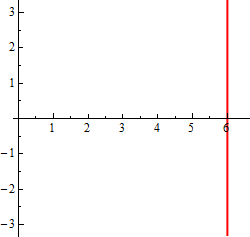Paul's Online Notes
Home / Calculus II / Parametric Equations and Polar Coordinates / Polar Coordinates
Show Mobile Notice Show All Notes Hide All Notes
Mobile Notice
You appear to be on a device with a "narrow" screen width (i.e. you are probably on a mobile phone). Due to the nature of the mathematics on this site it is best views in landscape mode. If your device is not in landscape mode many of the equations will run off the side of your device (should be able to scroll to see them) and some of the menu items will be cut off due to the narrow screen width.

### Section 9.6 : Polar Coordinates

9. Sketch the graph of the following polar equation.

$\cos \theta = \frac{6}{r}$ Show Solution

Multiplying both sides by $$r$$ gives,

$r\cos \theta = 6$

and we know from the notes on this section that this is simply the vertical line $$x = 6$$. So here is the graph of this function.